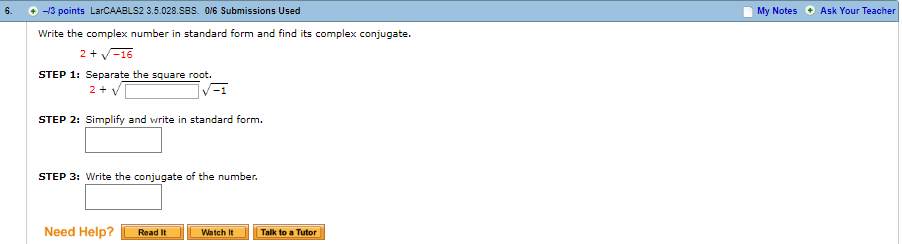# -13 points LarCAABL52 3.5.028.SBS. 016 Submissions UsedMy Notes Ask Your TeacherWrite the complex number in standard form and find its complex conjugate.2V16STEP 1: Separate the square root.STEP 2: Simplify and write in standard formSTEP 3: Write the conjugate of the number.Need Help?Read ItWatch t Talk to a Tutor

Question
6 views

6.1,help_outlineImage Transcriptionclose-13 points LarCAABL52 3.5.028.SBS. 016 Submissions Used My Notes Ask Your Teacher Write the complex number in standard form and find its complex conjugate. 2V16 STEP 1: Separate the square root. STEP 2: Simplify and write in standard form STEP 3: Write the conjugate of the number. Need Help?Read ItWatch t Talk to a Tutor fullscreen
check_circle

Step 1

To simplify the given complex number and express it in the usual notation

Step 2

The standard form of a complex number z is z=x+iy, where x and y are real numbers. The operations on complex numbers are identical as for the real numbers, except that the symbol i satisfies i^2=-1. Square roots are also handled in the same way as in the display.

Step 3

Given a complex number z in the standard form z=x+iy (x and y are real), the ...

### Want to see the full answer?

See Solution

#### Want to see this answer and more?

Solutions are written by subject experts who are available 24/7. Questions are typically answered within 1 hour.*

See Solution
*Response times may vary by subject and question.
Tagged in

### Complex Numbers